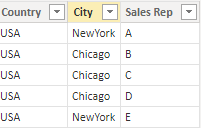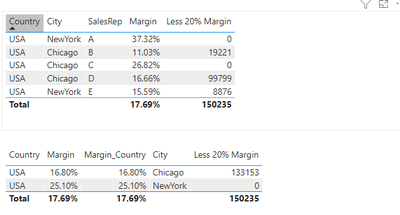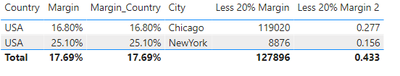cancel
Showing results for
Did you mean:

Fabric is Generally Available. Browse Fabric Presentations. Work towards your Fabric certification with the Cloud Skills Challenge.Helper I

## How to apply a measure calculation before roll up

Hello,

I needed some help to understand how to apply a DAX expression before roll up -

Here's a sample dataset -

DIMFACTI am trying to calculate the total sales amount from every city where the sales rep margin <20%. DAX works fine when I keep Sales Rep in the context but when I remove Sales rep field, the calculation doesnt work anymoreHere's my DAX

Margin = (Sum('Fact'[Selling])-sum('Fact'[Cost]))/sum('Fact'[Cost])
Less 20% Margin = IF([Margin]<.2,sum('Fact'[Selling]),0)

Appreciate any help with this.

Thanks
2 ACCEPTED SOLUTIONSSuper User

Add an iterator at whatever the appropriate level of granularity is.

Assuming Sales Rep the granularity you want, try this:

``````Less 20% Margin =
SUMX (
VALUES ( 'dim'[Sales Rep] ),
IF (
[Margin] < .2,
CALCULATE ( SUM ( 'Fact'[Selling] ) ),
0
)
)``````Super User

This measure is designed to add up the values for each Sales Rep separately so it shouldn't be used when you don't want to do that.

I'm not sure, but I'm guessing you want something more like this:

``````Less 20% Margin 2 =
CALCULATE (
[Margin],
FILTER (
VALUES ( 'dim'[Sales Rep] ),
[Margin] < .2
)
)``````
4 REPLIES 4Super User

Add an iterator at whatever the appropriate level of granularity is.

Assuming Sales Rep the granularity you want, try this:

``````Less 20% Margin =
SUMX (
VALUES ( 'dim'[Sales Rep] ),
IF (
[Margin] < .2,
CALCULATE ( SUM ( 'Fact'[Selling] ) ),
0
)
)``````Helper I

Thank you that works!!

I further changed the DAX to calculate margins of the sales reps <.2 and it works however the sub total row aggregates the percentages. Is there anyway to handle this please?

Less 20% Margin 2 =
SUMX(
VALUES ( 'dim'[Sales Rep] ),
IF (
[Margin] < .2,
CALCULATE ( [Margin]) ,
0
)
)ThanksSuper User

This measure is designed to add up the values for each Sales Rep separately so it shouldn't be used when you don't want to do that.

I'm not sure, but I'm guessing you want something more like this:

``````Less 20% Margin 2 =
CALCULATE (
[Margin],
FILTER (
VALUES ( 'dim'[Sales Rep] ),
[Margin] < .2
)
)``````Super User

Hi,

Without the sales rep context your calculation is done to all of the data in the data set and it seems for new york this aggregate is greater than 20%. To solve this you can calculate the margin in a calcuated column and then appy the if condition to the calculated column to get the margin on a transaction level.

I hope this helps to solve your issue and if it does consider accepting this as a solution.

Proud to be a Super User!Announcements#### Power BI Monthly Update - November 2023

Check out the November 2023 Power BI update to learn about new features.#### Fabric Community News unified experience

Read the latest Fabric Community announcements, including updates on Power BI, Synapse, Data Factory and Data Activator.#### The largest Power BI and Fabric virtual conference

130+ sessions, 130+ speakers, Product managers, MVPs, and experts. All about Power BI and Fabric. Attend online or watch the recordings.Top Solution Authors
Top Kudoed Authors
Users online (3,084)